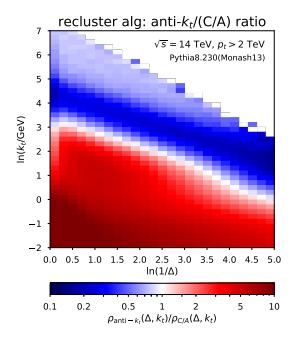# Setting Color Palette for TH2D

I am having trouble setting the color palette for a TH2D. I am only mildly familiar with making my own ColorGradientTable and I am having trouble creating one for the specifications I want. I want to create one that shows bin values less than 1 blue, shaded appropriately, and then values greater than 1 red, also shaded appropriately. I also would like to set the color of bins equal to 1 as white. I think I just don’t understand how to set the Length and RGB arrays appropriately to achieve this.

I.E. I am not sure how to set the following code to achieve this result:
const Int_t Number = 3;

Double_t Red[Number] = { 1.00, 0.00, 0.00};

Double_t Green[Number] = { 0.00, 1.00, 0.00};

Double_t Blue[Number] = { 1.00, 0.00, 1.00};

Double_t Length[Number] = { 0.00, 0.50, 1.00 };

Int_t nb=50;

I want it to look like the following:Any help would be appreciated!

Derek

``````{
TH2F *h1 = new TH2F("h1","h1",100,-4,4,100,-4,4);
h1->SetStats(0);

Double_t a,b;
for (Int_t i=0;i<50000;i++) {
gRandom->Rannor(a,b);
h1->Fill(a,b,0.16);
}

Double_t max = h1->GetMaximum();
Double_t min = h1->GetMinimum();
Double_t vw  = 5.;
Double_t pw  = (vw-min)/(max-min);
Double_t e   = 0.005;
Double_t l   = 0.9;

const Int_t Number = 6;
Double_t Red[Number]   = { 0.0, l  , 1., 1., 1.0, 1.0};
Double_t Green[Number] = { 0.0, l  , 1., 1., l  , 0.0};
Double_t Blue[Number]  = { 1.0, 1.0, 1., 1., l  , 0.0};
Double_t Stops[Number] = { 0., pw-e, pw-e, pw+e, pw+e, 1. };

Int_t nb= 256;
h1->SetContour(nb);

In the macro I sent you change the value of `vw` to 1.